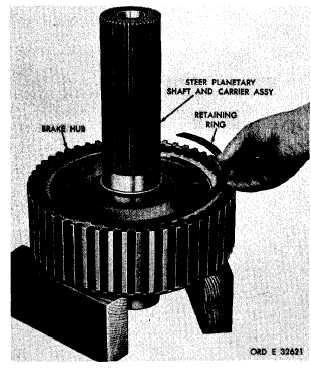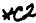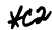Section 1 5 7 .   D E S C R I P T I O N a p .   Install   the   brake   coolant   pump   idler gear   shaft   and   secure   with   one   3/8-16   x   3-1/4 b o l t   ( f i g .   1 7 6 ) .   T o r q u e   t h e   b o l t   t o   2 6 - 3 2 p o u n d - f e e t. a q .   Install   the   bearing   in   the   brake   pump idler   gear   and   secure   with   the   snap   ring   (fig. 176). a r.   Install   the   idler   gear   with   the   bearing onto   the   shaft   and   secure   it   with   the   snap   ring (fig.    176). X I X .   L E F T -   A N D   R I G H T - S T E E R   P L A N E T A R I E S  R E B U I LD
Custom Search*C2 *C2 PAR  1 5 6 - 1 58 LEFT, RIGHT STEER PLANETARIES REBUILD C H A P   5,   SEC  X I X a .   Install   springs   and   washers   and   se- cure   with   pins   (fig.   200).   Bend   the   ends   of   the pins   to   retain   them. .   I n s t a l l    r e t a i n i n g    r i n g s ,    b r a k e    a ir v a l v e    p l a t e s    a n d    s p r i n g s    o n    b r a k e    a i r    v a l ve bracket   as   shown   (fig.   201). .   Install   right-   and   left-air   valve   plate assemblies   with   bracket   and   secure   with   two 3/8-16 self-locking bolts. Torque bolts to 36-43  pound-feet. Section 1 5 7 .    D E S C R I P T I O N .   Install   the   brake   coolant   pump   idler gear   shaft   and   secure   with   one   3/8-16   x   3-1/4 b o l t    ( f i g .    1 7 6 ) .    T o r q u e    t h e    b o l t    t o    2 6 - 3 2 p o u n d - f e e t. .   Install   the   bearing   in   the   brake   pump idler   gear   and   secure   with   the   snap   ring   (fig. 176). r.   Install   the   idler   gear   with   the   bearing onto   the   shaft   and   secure   it   with   the   snap   ring (fig.    176). X I X .    L E F T -    A N D    R I G H T - S T E E R    P L A N E T A R I E S — R E B U I LD .   R e m o v e    t h e    r e t a i n i n g    r i n g    f r o m    t he r i n g    g e a r    a s s e m b l y    ( f i g .    2 0 3 ). Refer   to   par.   16   for   the   description   of   the left-    and    right-steer    planetaries . 158.   DISASSEMBLY   (figs,   378   and   380, fold-outs   7   and   9) .   All   related   items   not   covered in     through  i,   below,   were   removed from   the   transmission   as   outlined   in par.   75,   steps   27   through   30   and   40 t h r o u g h    5 4 .    N o    f u r t h e r    d i s a s s e m b ly of   those   parts   is   required.   The   left- and    right-    steer    planetary    assemblies are    identical;    therefore,    the    follow- ing   disassembly   steps   apply   to   both a s s e m b l i e s. .   Remove   the   retaining   ring   that   retains the   steer   planetary   shaft   and   carrier   assembly in   the   brake   hub   (fig.   202). .   Remove   the   steer   planetary   shaft   and c a r r i e r    a s s e m b l y    ( f i g .    2 0 2 ) . .   Remove   the   steer   planetary   ring   gear from   the   brake   hub   (fig.   203). .   R e m o v e    t h e    t h r u s t    w a s h e r    f r o m    t he ring   gear   assembly   (fig.   203). .   P r e s s    o r    d r i v e    t h e    b e a r i n g    o u t    o f    t he b r a k e     h u b     ( f i g .     2 0 3 ). Figure 202. Removing (or installing) steer planetary carrier   and   shaft   retaining   ring 1 3 9Integrated Publishing, Inc. - A (SDVOSB) Service Disabled Veteran Owned Small Business